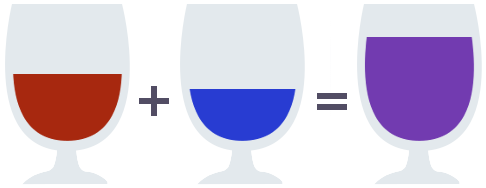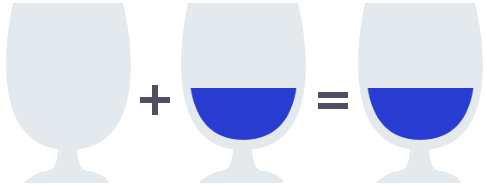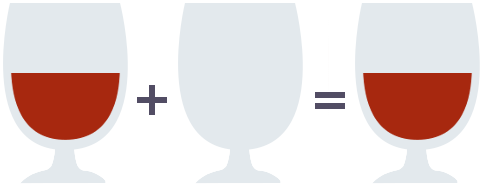Algebraic patterns — Identity element

Posted on June 29, 2016

Introduction

The Identity element pattern is all about defining the concept of emptiness, and is a good introduction to algebraically modelling programming concepts.

There are certain values — probably found scattered around the code you’re working on right now — values like the numbers 1 and 0, the empty string "" or the empty array [], that are often used as initializers and somehow feel “empty” or uninteresting.

The identity element pattern allows us to formalize this notion in a precise way, letting us explain just why these values are so common. That is, in a given code base, you’re probably more likely to see the string "", than say, the string "banana", and the number 0 more than the number 4279. Is there some way we can explain what makes these values special?

Imagine an empty glass. How can we define what it means for a glass to be empty? Now, of course, it is easy to do so informally, I just asked you to visualize one. But what is a formal property the empty glass satisfies, that non-empty glasses do not?

The key idea here will be to define an additional operation to combine two glasses. We will call this operation pour, and define it as taking two glasses and pouring their contents into a new identical container.Equipped with this operation, we can find a property fulfilled by the empty glass and the empty glass alone: Pouring the contents of the empty glass into another glass leaves the other glass unchanged.and similarly, pouring the contents of any glass into the empty glassFormally, we can write this as the following pair of equations, where x represents any arbitrary glass.

pour(emptyGlass, x) = x
pour(x, emptyGlass) = x

Definition

We can now take a look our other “empty” values, and check if they satisfy similar properties. And in fact, we will see that they do.

Take the number 0. To say that 0 is in our sense empty, we will need to find an operation such that the equations above hold. We will take the operation add, which adds two numbers together, i.e. the operation such that add(3, 9) = 12. Now, it’s clear that

so the value 0 is in fact empty according to our definition. In particular we say that 0 is the identity element with respect to the addition operation.

What about the empty array, or the empty string? We’ll kill two birds with one stone. Define the operation concat, such that concat("foo", "bar") = "foobar", and concat([1,2,3], [4,5]) = [1,2,3,4,5]. Then

concat("", x) = x
concat(x, "") = x

and

concat([], x) = x
concat(x, []) = x

The empty array, and the empty string, are identity elements with respect to their concatenation operations, and so their emptiness can be formally described.

In general we say that for a set A with a closed binary operation ⊕, an empty (identity) element e is an element in A such that for all x in A we have

x ⊕ e = x
e ⊕ x = x

We could go on all day finding examples of identity elements, they are quite common, and there are plenty hiding in the wild. Let’s look at couple more.

First, let’s continue in the domain of numbers. We’ll take another special value: infinity, or . Infinity might seems like the opposite of “empty”, but it will turn out to be an example of an identity element. How exactly can we say that infinity is an empty element of the numbers?

To understand this, think of upper bounds. Let’s say I make the statement, “The oldest person in the room is at most 80 years old”. This means I claim to have some information about how old the people in the room are. But what if I had absolutely no clue? One way to express this could be saying “The oldest person in the room is at most ∞ years old”.

Infinity, seen as an upper bound, conveys no information, and is thus in this sense empty. What operation should we choose to express this notion? The answer is to use the min operator.

min(∞, x) = x;
min(x, ∞) = x;

Exercise Make a similar argument for negative infinity, and choose a suitable operation to prove it’s “emptiness”.

Note that this means that for a given domain, multiple empty objects may exist, with different choices of operators to give meaning to a specific sense of emptiness. Another value we can choose is 1 with the operation mul, such that mul(5,3) = 15.

mul(1, x) = x;
mul(x, 1) = x;

This interpretation of emptiness is combinatorial in nature.

Another important example is the identity function, (x => x), or id as it’s often called. The identity function is the function that takes an input and returns it unchanged. That is id(3) = 3, or generally, id(x) = x

What operation could we choose to see that this is an identity element? The operators we’re interested in are usually some natural way of composing two elements, so the answer of course, is to choose function composition: (f, g) => x => f(g(x)), or as it is often written, the operator ∘. We then get the equations

f ∘ id = f
id ∘ f = f

This simply states that to process either the input or the output of a function by applying the identity function is a no-op.

If you ever wondered where the identity function derives its name from, we see it is because it’s an identity element with respect to function composition .

Applications

The key to making practical use of the identity element pattern is usually to simply recognize that such an element exists, or on occasion choosing a model so that such an element can be found, for instance using forests over trees.

Consider the build system gulp, where we can describe a build step to minify our code base in production mode only with the following definition.

const optimize = env.production
? minify()
: util.noop();